# sklearn.metrics.confusion_matrix¶

sklearn.metrics.confusion_matrix(y_true, y_pred, labels=None, sample_weight=None)[source]

Compute confusion matrix to evaluate the accuracy of a classification

By definition a confusion matrix $$C$$ is such that $$C_{i, j}$$ is equal to the number of observations known to be in group $$i$$ but predicted to be in group $$j$$.

Thus in binary classification, the count of true negatives is $$C_{0,0}$$, false negatives is $$C_{1,0}$$, true positives is $$C_{1,1}$$ and false positives is $$C_{0,1}$$.

Read more in the User Guide.

Parameters: y_true : array, shape = [n_samples] Ground truth (correct) target values. y_pred : array, shape = [n_samples] Estimated targets as returned by a classifier. labels : array, shape = [n_classes], optional List of labels to index the matrix. This may be used to reorder or select a subset of labels. If none is given, those that appear at least once in y_true or y_pred are used in sorted order. sample_weight : array-like of shape = [n_samples], optional Sample weights. C : array, shape = [n_classes, n_classes] Confusion matrix

References

  Wikipedia entry for the Confusion matrix (Wikipedia and other references may use a different convention for axes)

Examples

>>> from sklearn.metrics import confusion_matrix
>>> y_true = [2, 0, 2, 2, 0, 1]
>>> y_pred = [0, 0, 2, 2, 0, 2]
>>> confusion_matrix(y_true, y_pred)
array([[2, 0, 0],
[0, 0, 1],
[1, 0, 2]])

>>> y_true = ["cat", "ant", "cat", "cat", "ant", "bird"]
>>> y_pred = ["ant", "ant", "cat", "cat", "ant", "cat"]
>>> confusion_matrix(y_true, y_pred, labels=["ant", "bird", "cat"])
array([[2, 0, 0],
[0, 0, 1],
[1, 0, 2]])


In the binary case, we can extract true positives, etc as follows:

>>> tn, fp, fn, tp = confusion_matrix([0, 1, 0, 1], [1, 1, 1, 0]).ravel()
>>> (tn, fp, fn, tp)
(0, 2, 1, 1)


## Examples using sklearn.metrics.confusion_matrix¶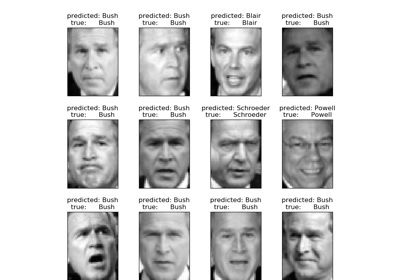Faces recognition example using eigenfaces and SVMs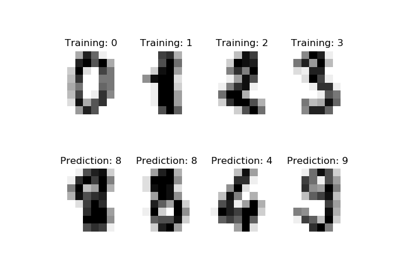Recognizing hand-written digits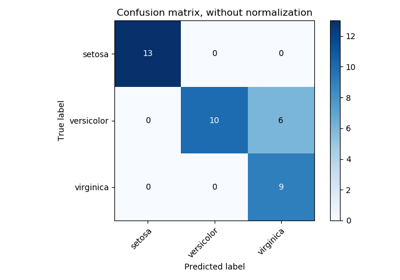Confusion matrix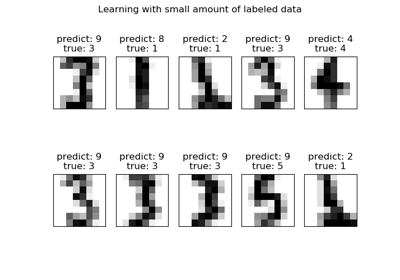Label Propagation digits: Demonstrating performance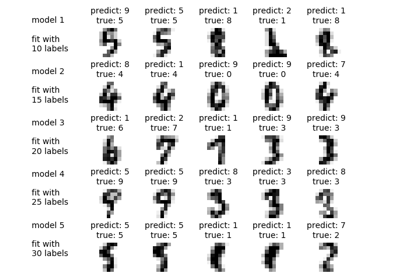Label Propagation digits active learning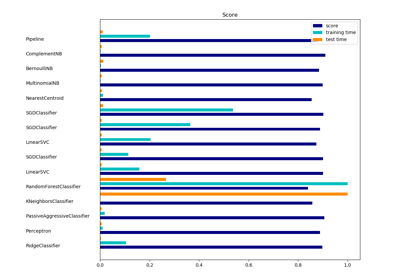Classification of text documents using sparse features